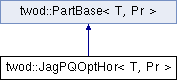# twod::JagPQOptHor< T, Pr > Class Template Reference

an optimal PxQ-way jagged partitionning algorithm using a Dynamic Programming based algorithm. More...

`#include <twod/jagged_dp_pq.hpp>`

Inheritance diagram for twod::JagPQOptHor< T, Pr >:## Detailed Description

### template<typename T, typename Pr> class twod::JagPQOptHor< T, Pr >

an optimal PxQ-way jagged partitionning algorithm using a Dynamic Programming based algorithm.

It is inspired from the algorithm in "Fredrik Manne and Tor Sorevik, Partitioning an Array onto a Mesh of Processors, PARA 1996". It takes the first dimension as the main dimension.

Parameters:
 T type of the load Pr type of the prefix sum array

List of all members.

## Public Member Functions

JagPQOptHor ()
void setP (int P)
selects the number of stripes in the main dimension.
virtual T part (int procCount, const Pr &prefixSumArray, util::RectList< T, Pr > &parts)
virtual ~JagPQOptHor ()

## Static Private Member Functions

static T jagged_dp_pq_internal (int P, int Q, const Pr &prefixSumArray, util::RectList< T, Pr > &parts)

int P

## Constructor & Destructor Documentation

template<typename T, typename Pr>
 twod::JagPQOptHor< T, Pr >::JagPQOptHor ( ) ` [inline]`
template<typename T, typename Pr>
 virtual twod::JagPQOptHor< T, Pr >::~JagPQOptHor ( ) ` [inline, virtual]`

## Member Function Documentation

template<typename T , typename Pr >
 T twod::JagPQOptHor< T, Pr >::jagged_dp_pq_internal ( int P, int Q, const Pr & prefixSumArray, util::RectList< T, Pr > & parts ) ` [static, private]`
template<typename T , typename Pr >
 T twod::JagPQOptHor< T, Pr >::part ( int procCount, const Pr & prefixSumArray, util::RectList< T, Pr > & parts ) ` [virtual]`

Applies 2D jagged-optimal partitioning. The partition ends up with P rows that has Q processor assigned to each row.

Parameters:
 [in] procCount is the number of processors [in] prefixSumArray first column and first row consists of zero only. But the borders of rectangles in rect_list never touch this area (index 0 in row or column) [out] parts must be allocated before calling this function. List of rectangles that form the partition.
Returns:

Implements twod::PartBase< T, Pr >.

template<typename T, typename Pr>
 void twod::JagPQOptHor< T, Pr >::setP ( int P ) ` [inline]`

selects the number of stripes in the main dimension.

Parameters:
 P number of stripes in the first dimension. If 0, the number of stripes will be computed at runtime by taking the square root of the number of processors.

## Member Data Documentation

template<typename T, typename Pr>
 int twod::JagPQOptHor< T, Pr >::P` [private]`

The documentation for this class was generated from the following files: